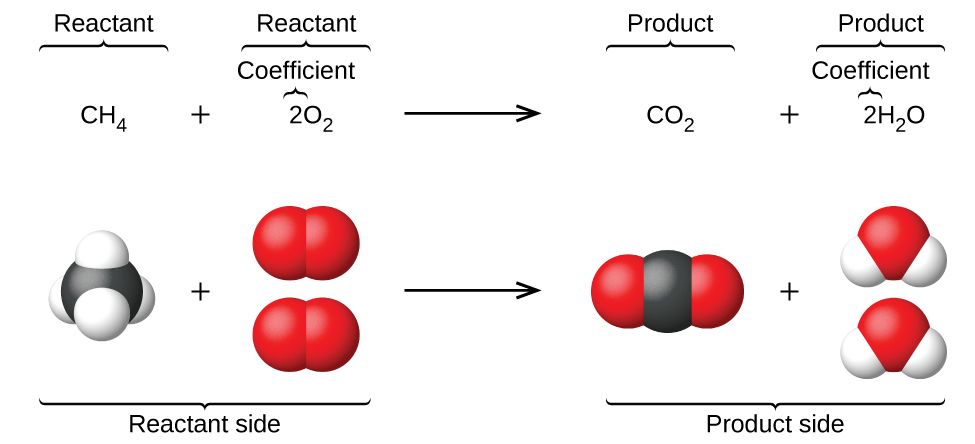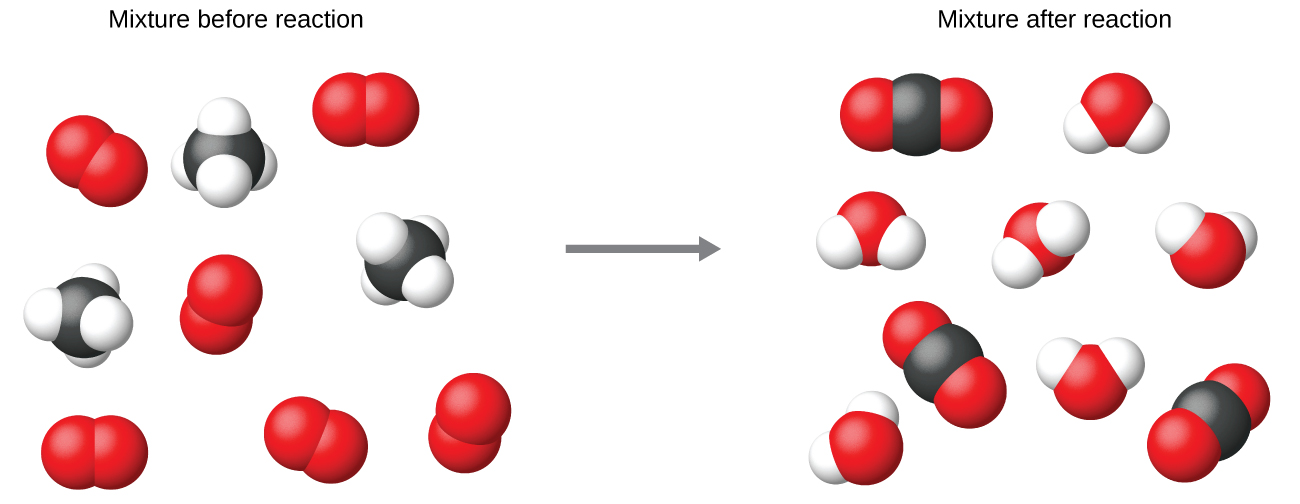4.1 Writing and balancing chemical equations

 Page 1 / 14
By the end of this section, you will be able to:
• Derive chemical equations from narrative descriptions of chemical reactions.
• Write and balance chemical equations in molecular, total ionic, and net ionic formats.

The preceding chapter introduced the use of element symbols to represent individual atoms. When atoms gain or lose electrons to yield ions, or combine with other atoms to form molecules, their symbols are modified or combined to generate chemical formulas that appropriately represent these species. Extending this symbolism to represent both the identities and the relative quantities of substances undergoing a chemical (or physical) change involves writing and balancing a chemical equation    . Consider as an example the reaction between one methane molecule (CH 4 ) and two diatomic oxygen molecules (O 2 ) to produce one carbon dioxide molecule (CO 2 ) and two water molecules (H 2 O). The chemical equation representing this process is provided in the upper half of [link] , with space-filling molecular models shown in the lower half of the figure.The reaction between methane and oxygen to yield carbon dioxide and water (shown at bottom) may be represented by a chemical equation using formulas (top).

This example illustrates the fundamental aspects of any chemical equation:

1. The substances undergoing reaction are called reactants , and their formulas are placed on the left side of the equation.
2. The substances generated by the reaction are called products , and their formulas are placed on the right sight of the equation.
3. Plus signs (+) separate individual reactant and product formulas, and an arrow $\text{(⟶)}$ separates the reactant and product (left and right) sides of the equation.
4. The relative numbers of reactant and product species are represented by coefficients (numbers placed immediately to the left of each formula). A coefficient of 1 is typically omitted.

It is common practice to use the smallest possible whole-number coefficients in a chemical equation, as is done in this example. Realize, however, that these coefficients represent the relative numbers of reactants and products, and, therefore, they may be correctly interpreted as ratios. Methane and oxygen react to yield carbon dioxide and water in a 1:2:1:2 ratio. This ratio is satisfied if the numbers of these molecules are, respectively, 1-2-1-2, or 2-4-2-4, or 3-6-3-6, and so on ( [link] ). Likewise, these coefficients may be interpreted with regard to any amount (number) unit, and so this equation may be correctly read in many ways, including:

• One methane molecule and two oxygen molecules react to yield one carbon dioxide molecule and two water molecules.
• One dozen methane molecules and two dozen oxygen molecules react to yield one dozen carbon dioxide molecules and two dozen water molecules.
• One mole of methane molecules and 2 moles of oxygen molecules react to yield 1 mole of carbon dioxide molecules and 2 moles of water molecules.Regardless of the absolute numbers of molecules involved, the ratios between numbers of molecules of each species that react (the reactants) and molecules of each species that form (the products) are the same and are given by the chemical reaction equation.

the first fifty elements
how to complete and balance ionic equation?
what is molar mass?
Molar mass is defined as the mass of a sample of a compound divided by the amount of substance of that sample.
Love
right
xavi
or M=m\n mathematically.
xavi
atom is the smallest indivisible part of an element that takes place in chemical reaction
An atom is the smallest indivisible part of an element
Vibes
what is periodic table
Faridi
what is chemistry?
what is an atom
Mathias
what is an atom?
Mathias
is the study of matter and the changes it under goes.
xavi
under goes through three state they are solid liquid and gas
Samuel
in a sturctural way formula for C6H12
Samuel
sorry ,it's properties and structures
xavi
please what is hydroxyl group
the hydroxyl group is a functional group consisting of a hydrogen atom covalently bonded to an oxygen atom.
Itz
Names are of homologous series
what's an hydroxyl group?
A hydroxy or hydroxyl group is the entity with the formula OH. It contains oxygen bonded to hydrogen. In organic chemistry, alcohols and carboxylic acids contain hydroxy groups. The anion [OH⁻], called hydroxide, consists of a hydroxyl group.
okwara
what are the steps to follow when writing the condensed formula of elements
What is organic chemistry
what's organic chemistry?
why do electrons have no mass
hello
Samuel
hello there
Oliver
hi and who say electrons has no mass ? it does have but most at time consider negleable
Eyong
hello
Lagazani
please what is maa
Lagazani
yeah i will calculate it
divine
Because it's mass it very very small compare with mass of atom
Yasmeen
it's simple
lola
consider the rxn of butadiene .Butadiene is prepared by the gas phase catalytic dehydrogenation of 1-butene , at 900K and 1 bar. c4H8 (g)_ C4H6 (g) + H2 (g) (a) In order to suppress side reactions , the butane is diluted with steam before it passes into the reactor.Estimate the conversion
Rodrick
Estimate the conversion of 1-butene for a feed consisting of 10 moles of steam per mole of 1-butene. (b) find the conversion if the inerts were absent and side reactions are ignored .
Rodrick
help me please I have tried
Rodrick
wat is the difference between electrochemical chemical corrosion
Uzor
please how can i solve this project work..modern aid science process and product
Lagazani
what is the definition of chemistry
Sophia pls check on fb u will see transcendence Internationale is a group from here just join over, and ask any question in science from there we here created it
Eyong
the icon is clear white then writing transcendence Internationale study group
Eyong
U will see it
Eyong
for now chemistry is the study of matter and it properties, why and how element combined to form other substances
Eyong
Chemistry is a group that identifies properties of matter and many more
Alona
Wow and chemistry also is the systematic study of nature
Eyong
chemistry is the study of the structure, properties of matter and the effect of addition or removal of heat from substances.
Bra
calculate the relative molecular Mass of the following 1 ZnCl2 2 NaNo3 3 HNO3 4 CaO
2) 23+14+(16×3)= 3)1+14+(16×3)= 4) 40+16= 1) Zn+71= that's how they do it but for Zn I don't know its mass number
Eyong
Reuben u are welcome to join the group transcendence Internationale on fb we created it for science students is a question and answering platform for all science subjects
Eyong
yeah it's fun
Christian
hi guys I'm new here, please I need definition of acid and base
Gold
please some one should tell me the meaning of hydro carbon
Glory
radioactivity is the spontaneous disintegration of the nucleus of an atom of an element to emmit radiation
VivianByByBy